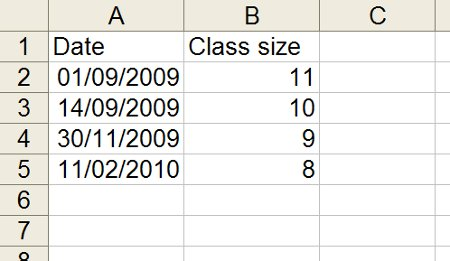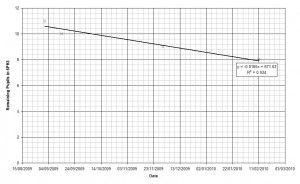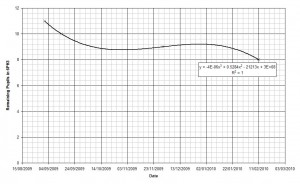# The dangers of extrapolation

Physics isn’t for everyone; every year there’s a pretty good chance that at least one person will drop out of a group (sometimes you get drop ins too). This year one group in particular has lost more pupils than average so I decided to start collecting data.I plotted the data and added a line of best fit.The R2 value is 0.934 so there’s fairly good correlation in the dataset. Now that I have an equation linking the date (x) and the number of pupils in the class (y) I can work out when the next pupil will leave the group by setting y to equal seven.

y = −0.0165x + 671.63
7 = −0.0165x + 671.63
x = 40280.61

Excel handles dates in a strange way, so the figure of 40280.61 needs to be converted into a regular date: now I know that the next pupil will leave 6PH3 on the 12th April 2010 (at half past two).

I can even find out when the class will be empty by solving for y=0.

0 = −0.0165x + 671.63
x = 40704.84

The class will finally empty out at 10th June 2011 at twenty past eight.

I thought that perhaps the linear fit wasn’t the best approach so I tried a polynomial fit, which turned out to have an R2 value of exactly 1.It turns out that this line only has one real solution, and according to that, I won’t run out of pupils until 27th June 2103.